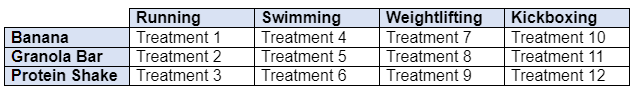## Q. What is the difference between factors, factor levels, and treatments?

Factor is another way of referring to a categorical variable. Factor levels are all of the values that the factor can take (recall that a categorical variable has a set number of groups). In a designed experiment, the treatments represent each combination of factor levels. If there is only one factor with k levels, then there would be k treatments. However, if there is more than one factor, then the number of treatments can be found by multiplying the number of levels for each factor together.

For example, say you have two factors, exercise type and post-exercise snack. Exercise type has four levels (running, swimming, weightlifting, kickboxing) and snack has three levels (banana, granola bar, protein shake). If we multiply 4 by 3, we get 12 treatments, which can be represented as follows:### Topics

• Last Updated Apr 16, 2021
• Views 0